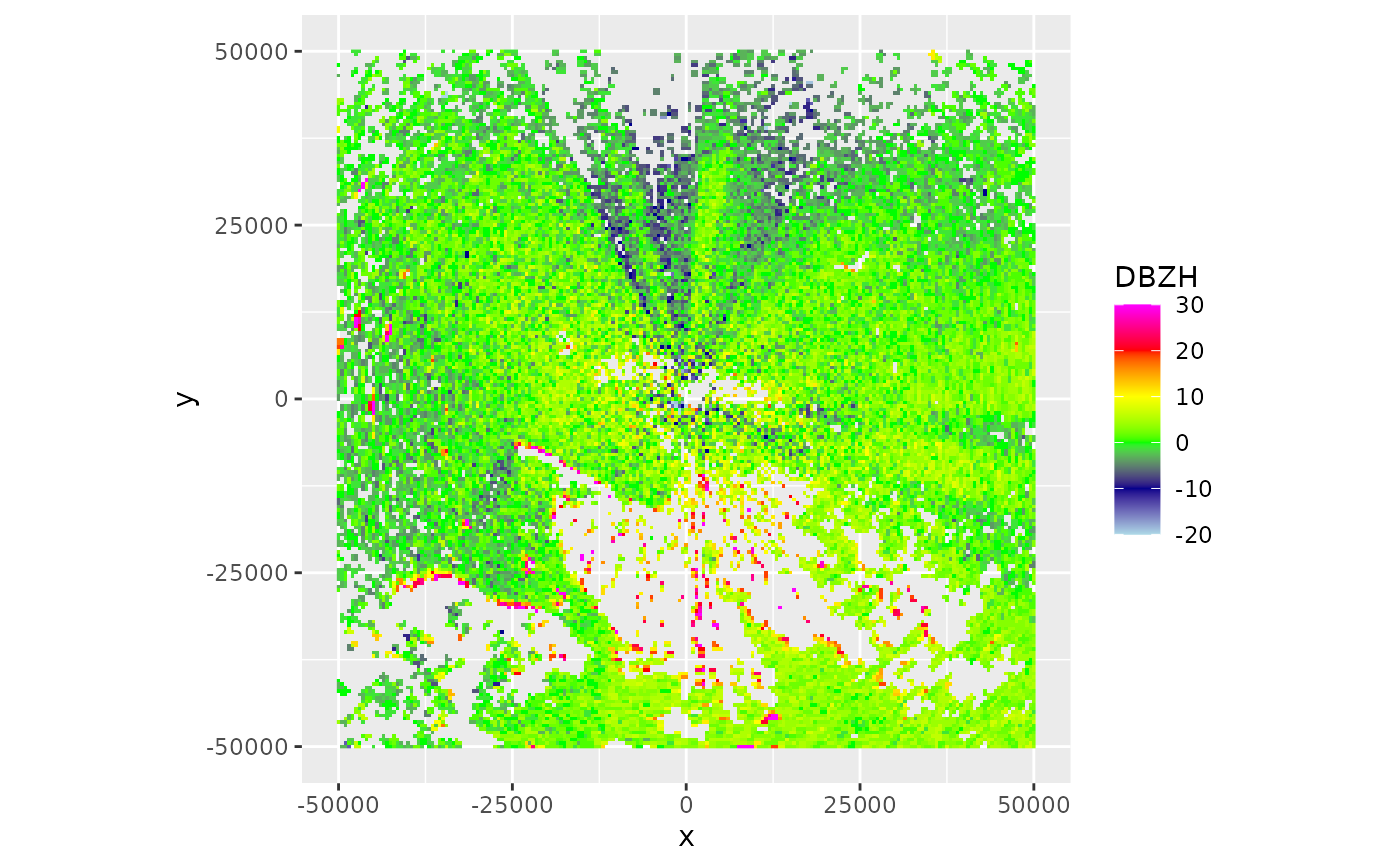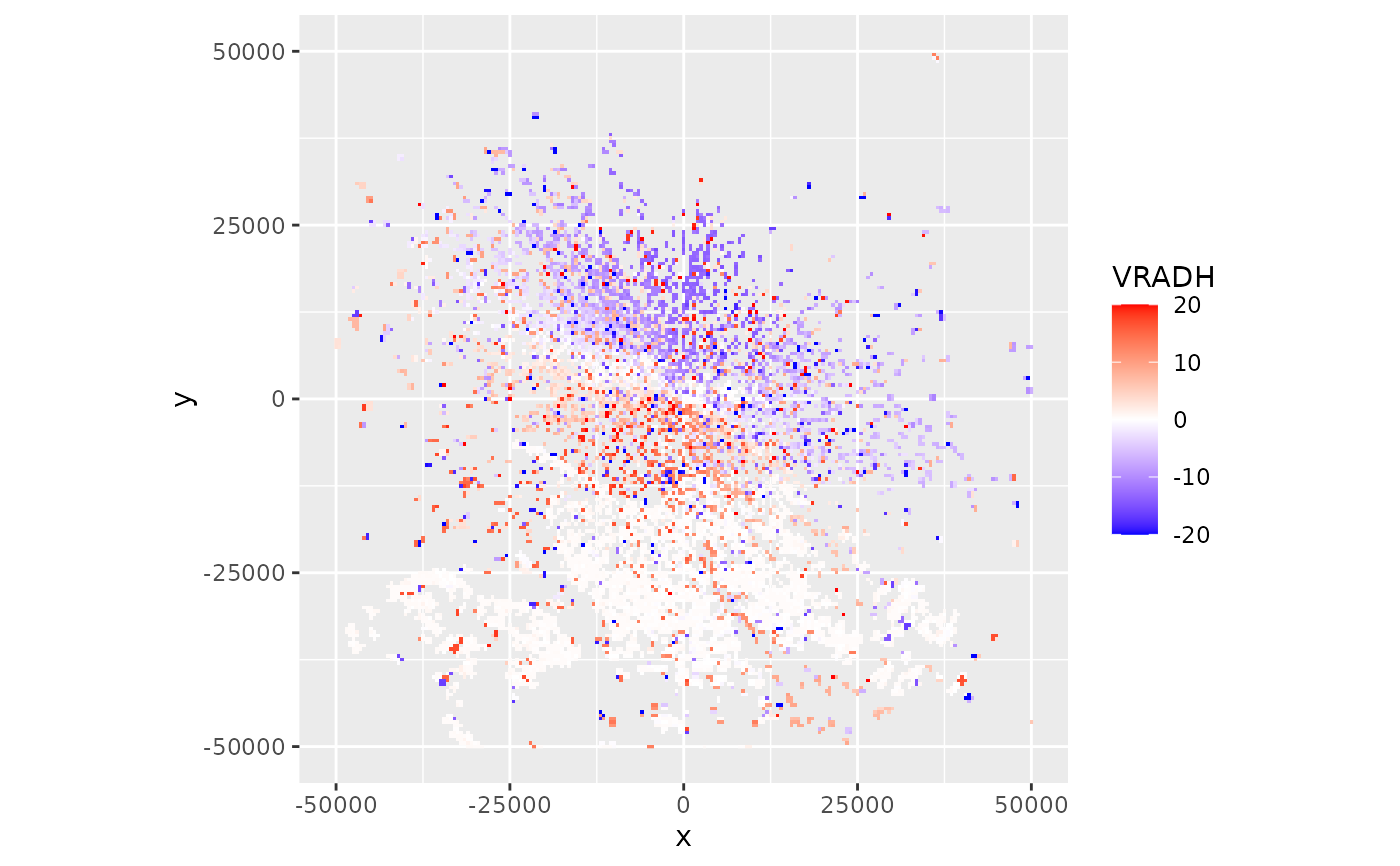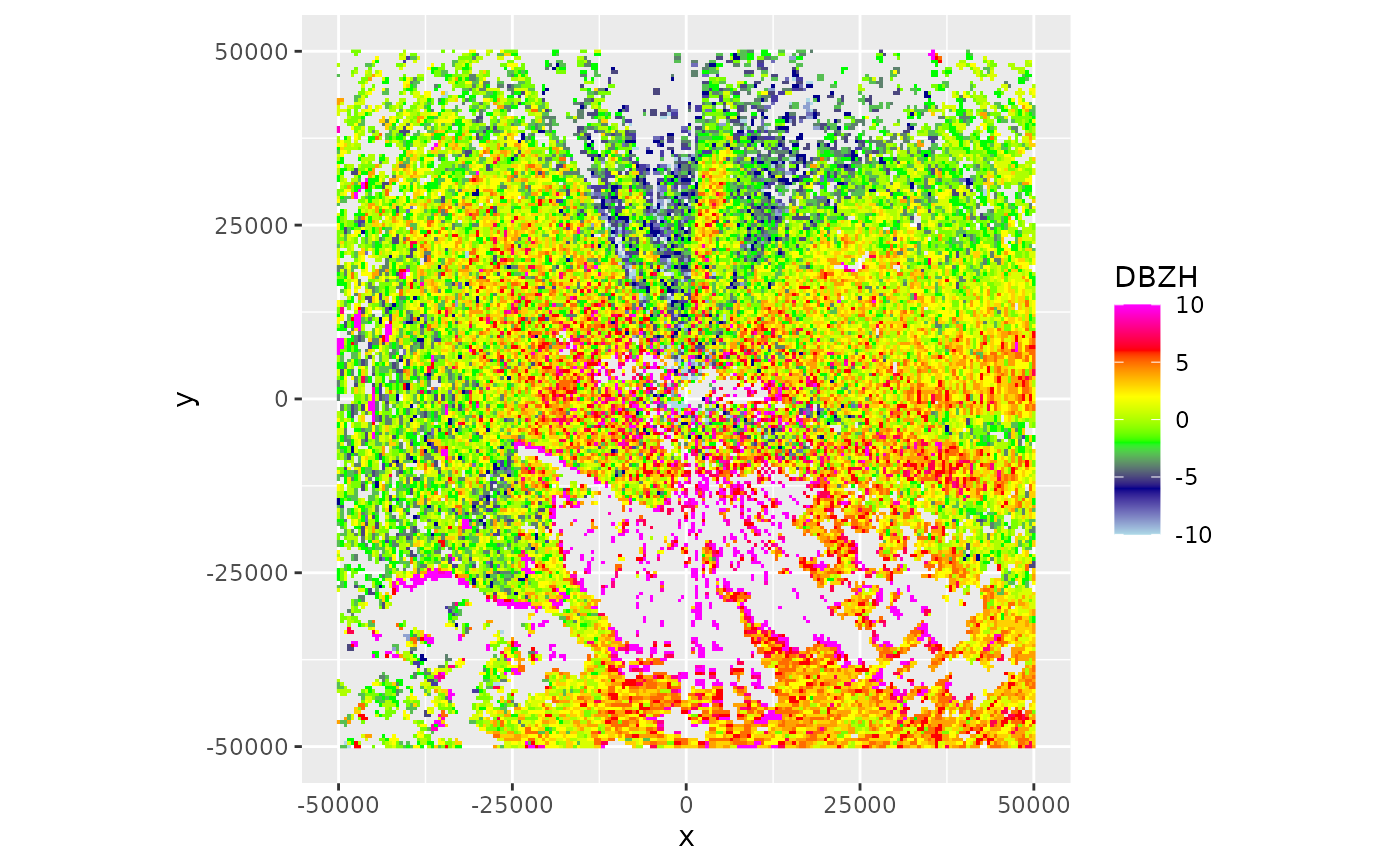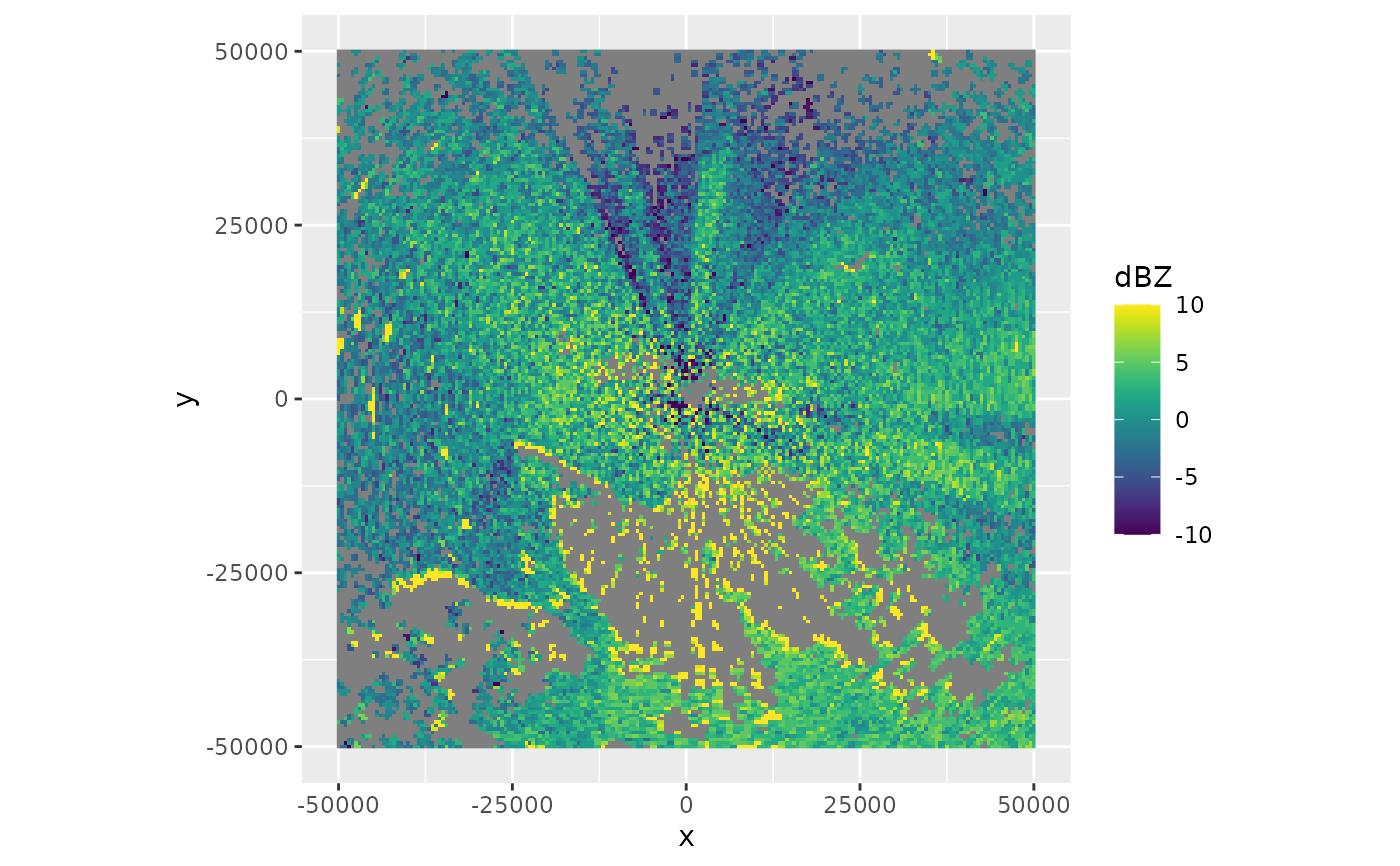Plot a plan position indicator (PPI) generated with project_to_ppi using ggplot

# S3 method for ppi
plot(
x,
param,
xlim,
ylim,
zlim = c(-20, 20),
ratio = 1,
na.value = "transparent",
...
)

## Arguments

x

An object of class ppi.

param

The scan parameter to plot, see details below.

xlim

Range of x values to plot.

ylim

Range of y values to plot.

zlim

The range of parameter values to plot.

ratio

Aspect ratio between x and y scale.

na.value

ggplot argument setting the plot color of NA values

...

Arguments passed to low level ggplot function.

## Details

Available scan parameters for plotting can by printed to screen by summary(x). Commonly available parameters are:

"DBZH", "DBZ"

(Logged) reflectivity factor (dBZ)

"TH", "T"

(Logged) uncorrected reflectivity factor (dBZ)

"VRADH", "VRAD"

"RHOHV"

Correlation coefficient (unitless). Correlation between vertically polarized and horizontally polarized reflectivity factor

"PHIDP"

Differential phase (degrees)

"ZDR"

(Logged) differential reflectivity (dB)

The scan parameters are named according to the OPERA data information model (ODIM), see Table 16 in the ODIM specification.

## Examples

# load an example scan:
data(example_scan)

# print to screen the available scan parameters:
summary(example_scan)
#>                   Polar scan (class scan)
#>
#>      parameters:  DBZH VRADH RHOHV ZDR PHIDP
#> elevation angle:  0.5 deg
#>            dims:  480 bins x 360 rays

# make ppi for the scan
ppi <- project_as_ppi(example_scan)
#> Warning: CRS object has comment, which is lost in output; in tests, see
#> https://cran.r-project.org/web/packages/sp/vignettes/CRS_warnings.html

# plot the default scan parameter, which is reflectivity "DBZH":
plot(ppi)# plot the radial velocity parameter: